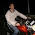### Water Jug Problem

Source: Introduction to Algorithms (by Cormen, Rivest, Leiserson)

Problem:
Suppose that you are given 'n' red and 'n' blue water jugs, all of different shapes and sizes. All red jugs hold different amounts of water, as do the blue ones. For every red jug, there is a blue jug that holds the same amount of water and vice versa.

How can you find the grouping of the jugs into pairs of red and blue jugs that hold the same amount of water, in the minimum number of comparisons.

The only operations allowed is compare between a red and a blue jar (no two reds, no two blues)

Update (Dec 30, 2010):
Problem statement changed a bit to make it more understandable.
Solution: Posted by Nikhil Garg (CSE, IIT Delhi third year undergraduate student) and Vivek Chaurasiya (Software Eng Symantec & CSE, IITR Alumnus) in comments! Explained in detail by me in comments!

1.Hint : Quick sort !

2.Agree with Nikhil.

red => r[1...n]
blue => b[1...n]

Take b[i] and Partition array r[] as in Quicksort using b[i] as pivot.

So, jug next to b[i] will have same size as of b[i].

Loop for i = [1....n] similarly.

Complexity O(n^2) ?

3.Agree with Nikhil.

red => r[1...n]
blue => b[1...n]

Take b[i] and Partition array r[] as in Quicksort using b[i] as pivot.

So, jug next to b[i] will have same size as of b[i].

Loop for i = [1....n] similarly.

Complexity O(n^2) ?

4.Yes, the algo is right. Its worst case order O(n^2) and average case O(nlog n)

Writing the solution in detail:

Let the algorithm be MATCHJUGS

MATCHJUGS(r[], b[])
{

Using b[i] as pivot, partition array r[]. This takes O(n).

r[] gets divided into r_small[] and r_large[] and element r_equal (equal to b[i])

Now, using r_equal as pivot, partition b[] into b_small[] and b_large[] and element b[i] (This takes another O(n))

Now you have r_small[], r_large[], b_small[], b_large[] with a total of 2(n-1) elements

Solve MATCHJUGS(r_small[], b_small[])
and MATCHJUGS(r_large[], b_large[]) recursively

}

This algorithm is analogous to quick sort and hence takes O(n logn) in average case and O(n^2) in worst case.